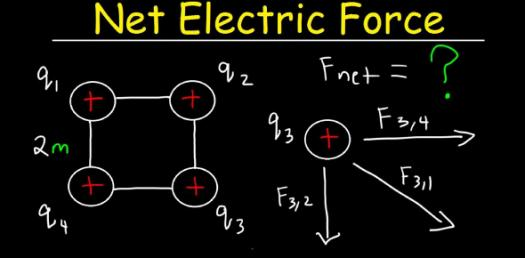# Quiz: Trivia Questions On Types Of Force!

15 Questions | Total Attempts: 325SettingsQuiz: Trivia Questions On Types Of Force! In physics class, you are introduced to the concept of force, and this means that it is the push or pull that is exerted on an object. There are different types of force, and the quiz below is perfect for seeing just how well you know them. Do give it a shot and see how well you will do.

Related Topics
• 1.
A combination of all the forces acting on an object is referred to as the
• A.

Net force

• B.

Absolute friction

• C.

Negative force

• D.

Speed

• 2.
The SI unit of measure for the strength fo a force is the _____
• A.

Newton

• B.

Einstein

• C.

Brown

• D.

Daltonian

• 3.
When we measured force in our labs we used a piece of lab equipment called a ___________
• A.

Spring scale

• B.

Triple beam balance

• C.

Doctor's scale

• D.

Rubber band

• 4.
What happens when two forces act in the same direction?
• A.

• B.

They cancel each other out

• C.

The stronger one prevails

• D.

Their sum divided by two is the net force

• 5.
Weight is the force of ____________ on an object at the surface of a planet (like Earth).
• A.

Gravity

• B.

Friction

• C.

Air resistance

• D.

Magnetism

• 6.
When the only force acting on a falling object is gravity, the object is said to be in _____
• A.

Free fall

• B.

Stationary

• C.

Deceleration

• D.

Good shape

• 7.
A push or a pull in science is referred to as a ___
• A.

Force

• B.

Inertia

• C.

Motion

• D.

Mass

• 8.
The force that results from two surfaces rubbing together is referred to as ________
• A.

Friction

• B.

Magnetism

• C.

Gravity

• D.

Elastic

• 9.
Two things determine the strength of friction, how hard the surfaces are pushed together and,,,
• A.

The types of surfaces involved

• B.

The spring scale you use

• C.

The temperature

• D.

The time of day

• 10.
Gravity is a force that ______ objects toward __________
• A.

Pulls; each other

• B.

Repels; the sun

• C.

Pushes; the poles

• D.

Separates; their partners

• 11.
Magnets stick to materials made out of...
• A.

• B.

Iron

• C.

Nickel

• D.

Cobalt

• 12.
Magnetic force surrounds a magnet and is caused by ...
• A.

Electric charges in the atoms that make up the magnet lining up in the same way

• B.

Magic

• C.

Tiny living cells that only live to put off a magnetic field

• D.

Pieces of a rock originally from outer space that crashed here many years ago

• 13.
If something returns to its original shape after it is squeezed or stretched it has .....
• A.

Elastic force

• B.

Magnetic force

• C.

Gravitational force

• D.

Friction force

• 14.
We know that the Earth acts as a large bar magnet because...
• A.

We see tiny magnetic minerals lined up to with the Earth's magnetic field in rocks of all ages

• B.

We asked it and there are people who can hear it answer

• C.

Scientists did a test in which they tried to repel a large bar magnet from the space station.

• D.

The north pole sticks up out of the ground near Norway and we can see it

• 15.
Compasses work because...
• A.

Its needle is a small magnet and it is pulled by Earth's magnetic field to point to the North Pole

• B.

It spins until you reach your destination at which time it will stop...so you know you are there

• C.

It knows where you want to go and it points you in that direction

• D.

If you know which way is north the compass will help you to walk that way